# Precalculus : Circles

## Example Questions

1 2 4 Next →

### Example Question #27 : Determine The Equation Of A Circle In Standard Form

Write the equation for a circle with a center at (5,6) and a radius of 2.

Possible Answers: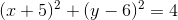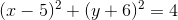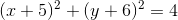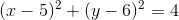Correct answer:Explanation:

The general equation for a circle is: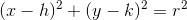The coordinates of the center of the circle is (h,k) and r is the radius.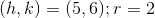So plugging in the coordinates the circle equations is: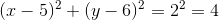### Example Question #28 : Determine The Equation Of A Circle In Standard Form

Find the equation of the circle in standard form.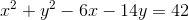Possible Answers: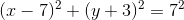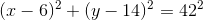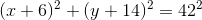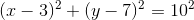Correct answer:Explanation:

The equation of a circle in standard form is given aswhere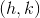is the coordinates of the center of the circle andis the length of the radius.

In order to get the equationin standard form we must complete the square.

First we group together similar variables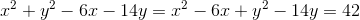Then we complete the square by

1. finding half of the coefficients of each variable with degree 1
2. squaring those results
3. and then adding those numbers to the equation.

As such,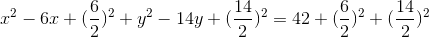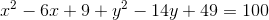And because the square of a difference is given as this equation through factoring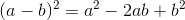we have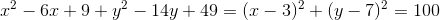or### Example Question #29 : Determine The Equation Of A Circle In Standard Form

Given the following equation for a circle, determine the coordinates of its center, as well as the coordinates of the four points directly, above, below, to the left, and to the right of the center: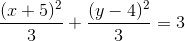Possible Answers: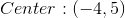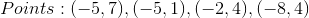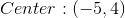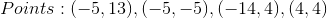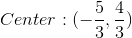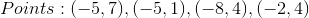Correct answer:Explanation:

First we must express the equation in standard form we can determine what the radius of our circle will be. The standard form for the equation of a circle is given as follows:Where the point (h,k) gives the center of the circle and r is the radius of the circle, which can be easily determined by taking the square root of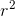once the equation is in standard form. Our first step is to multiply both sides of the equation by 3 to cancel the division by 3 on the left side: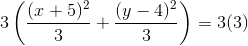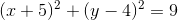Now we can see that our equation is in standard form, where h=-5 and k=4, which tells us the coordinates of the center of the circle:We can also determine the radius of the circle by taking the square root of: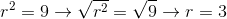Now that we know the center of the circle is at (-5,4), and that its radius is 3, we can find the points directly above and below the center by adding 3 to its y-coordinate, and then subtracting 3, respectively, giving us: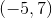and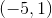Similarly, to find the points directly to the left and to the right of the center, we subtract 3 from its x-coordinate, and then add 3, respectively, giving us: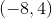and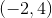### Example Question #30 : Determine The Equation Of A Circle In Standard Form

Graph the circle indicated by the equation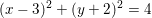Possible Answers: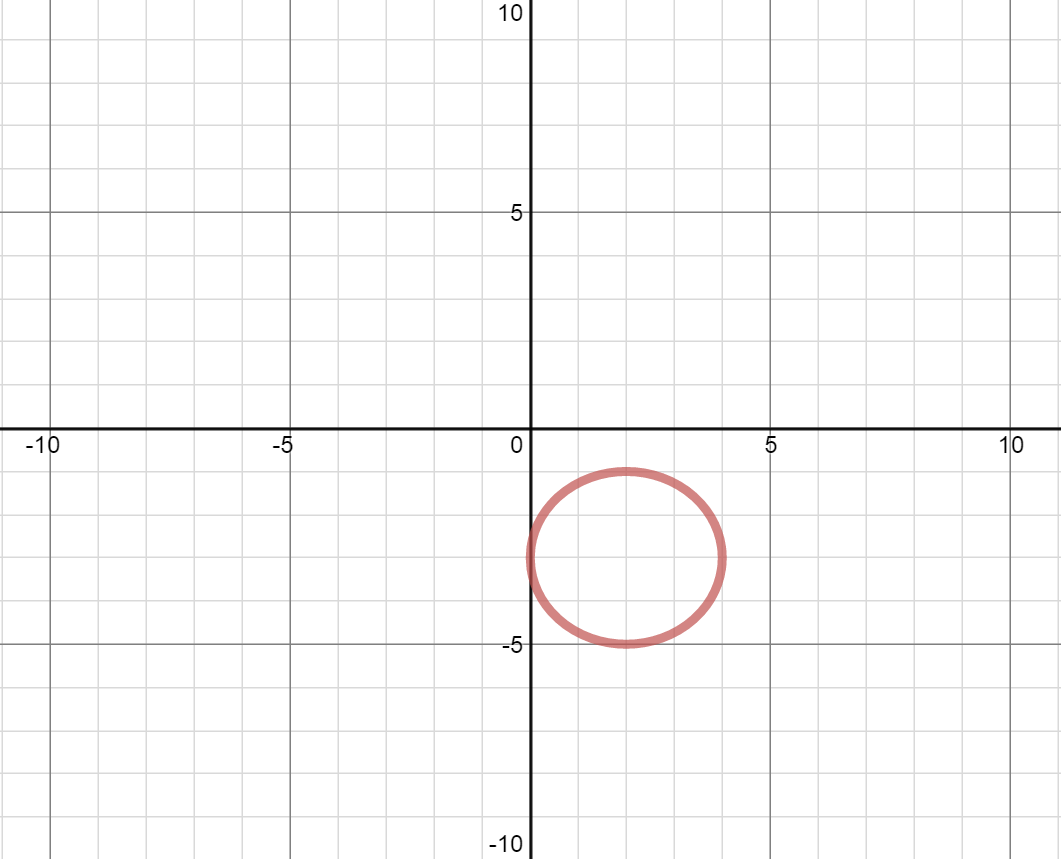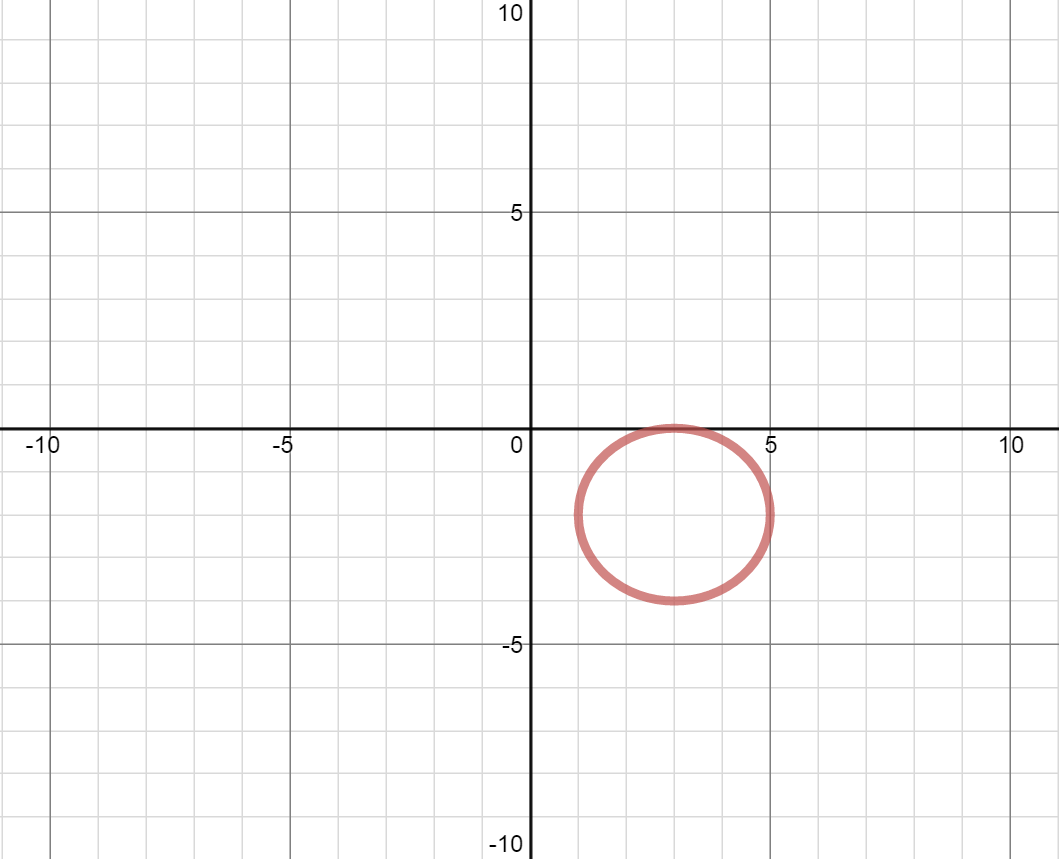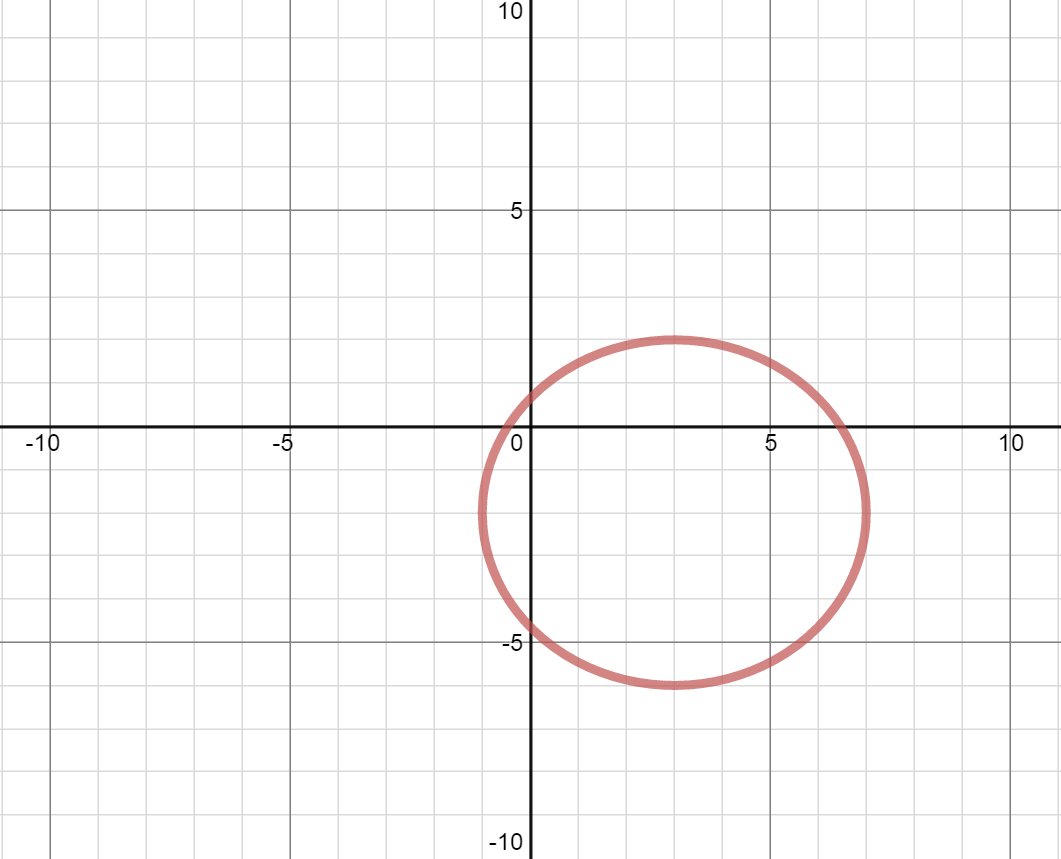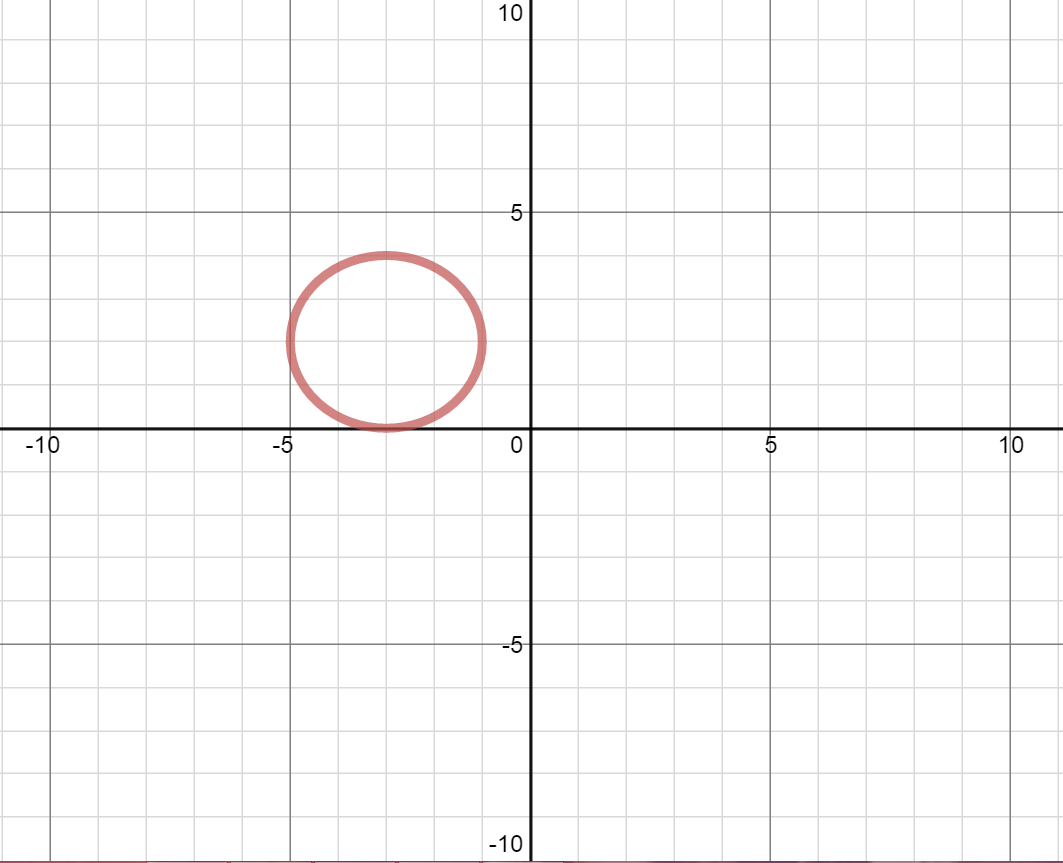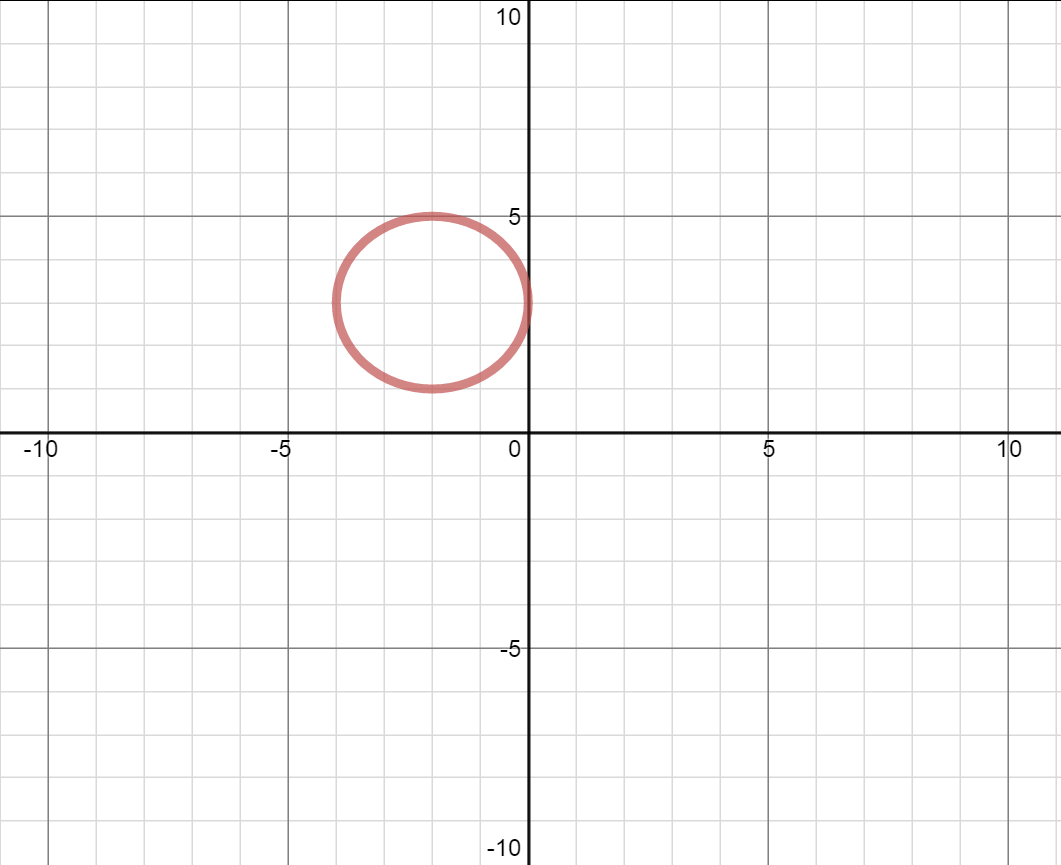Correct answer:Explanation:

We must begin by recalling the general formula for the equation of a circle.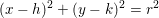Where circle has center of coordinates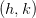and radius of.

That means that looking at our equation, we can see that the center is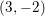.

If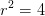, then taking the square roots gives us a radius of 2.

We then look at our possible choices.  Only two are centered at.  Of these two, one has a radius of 2 while one has a radius of 4.  We want the former.### Example Question #31 : Determine The Equation Of A Circle In Standard Form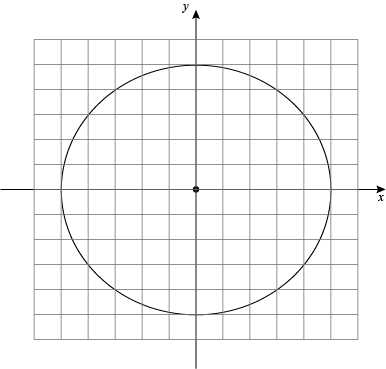If each mark on the graph representsunits, what is the equation of the circle?

Possible Answers: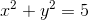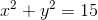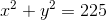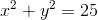Correct answer:Explanation:

Since the circle is centered at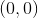we use the  most basic form for the equation for a circle: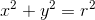.

Given the circle has a radius ofmarks, which representunits each, the circle has a radius ofunits.

We then plug infor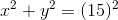and simplify:### Example Question #91 : Conic Sections

Which point is NOT on the circle defined by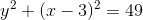?

Possible Answers: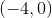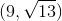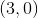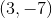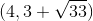Correct answer:Explanation:

The pointis the center of the circle - it is not on the circle.

We can test to see if the other points are actually on the circle by plugging in their x and y values into the equation. For example, to verify thatis actually on the circle, we can plug infor x and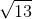for y: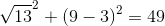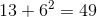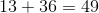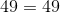this is true, so that point is on the circle.

### Example Question #33 : Determine The Equation Of A Circle In Standard Form

Which best describes the point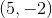and the circle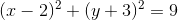?

Possible Answers:

The point is inside the circle

The point is on the circle

The point is outside the circle

The point is the focus of the circle

The point has no relationship with the circle

Correct answer:

The point is outside the circle

Explanation:

To quickly figure this out, we can plug in 5 for x and -2 for y and see what happens: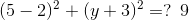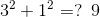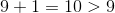Since the value is greater than 9, this point is outside the radius of this circle.

1 2 4 Next →

### All Precalculus Resources